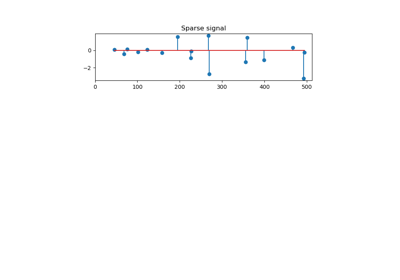# sklearn.datasets.make_sparse_coded_signal¶

sklearn.datasets.make_sparse_coded_signal(n_samples, n_components, n_features, n_nonzero_coefs, random_state=None)[source]

Generate a signal as a sparse combination of dictionary elements.

Returns a matrix Y = DX, such as D is (n_features, n_components), X is (n_components, n_samples) and each column of X has exactly n_nonzero_coefs non-zero elements.

Read more in the User Guide.

Parameters: n_samples : int number of samples to generate n_components : int, number of components in the dictionary n_features : int number of features of the dataset to generate n_nonzero_coefs : int number of active (non-zero) coefficients in each sample random_state : int, RandomState instance or None (default) Determines random number generation for dataset creation. Pass an int for reproducible output across multiple function calls. See Glossary. data : array of shape [n_features, n_samples] The encoded signal (Y). dictionary : array of shape [n_features, n_components] The dictionary with normalized components (D). code : array of shape [n_components, n_samples] The sparse code such that each column of this matrix has exactly n_nonzero_coefs non-zero items (X).

## Examples using sklearn.datasets.make_sparse_coded_signal¶Orthogonal Matching Pursuit